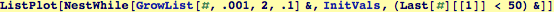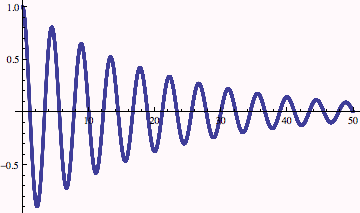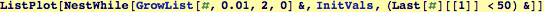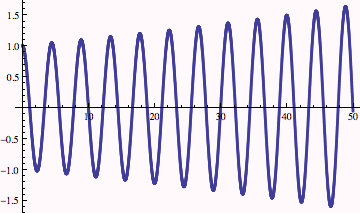Visualizing Second-Order Iteration

Visualize results out to x=50 (takes about 24 seconds to visualize )Change parameters for Growth Function (this example shows that the numerical solution does not converge to the accurate solution):Created by Wolfram Mathematica 6.0  (16 November 2007)